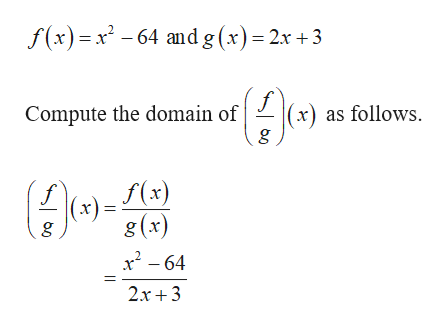# Find a specific domain and express it in interval notation For f(x)=x^2-64 and g(x)=2x+3, what is the domain of (f/g)(x)

Question
4 views

Find a specific domain and express it in interval notation
For f(x)=x^2-64 and g(x)=2x+3, what is the domain of (f/g)(x)

check_circle

Step 1

The given functi...help_outlineImage Transcriptionclosef(x)=x2 -64 and g (x) 2x +3 (x) as follows Compute the domain of f(x) f (x) g g (x) x2-64 2x3 fullscreen

### Want to see the full answer?

See Solution

#### Want to see this answer and more?

Solutions are written by subject experts who are available 24/7. Questions are typically answered within 1 hour.*

See Solution
*Response times may vary by subject and question.
Tagged in

### Other